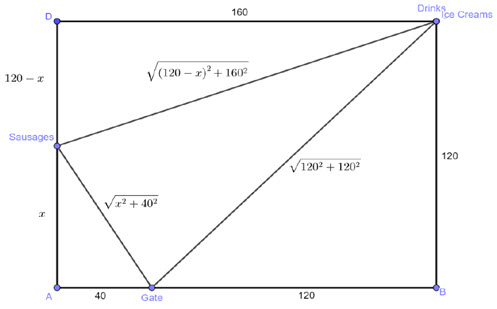Please note: This site contains links to websites not controlled by the Australian Government or ESA. More information here.

Go to website

## reSolve: Geometry: Lunch Lap (Trial)

In this sequence of two lessons, students apply Pythagoras' Theorem to explore a practical problem involving optimising paths to lunch carts. In the first lesson, students investigate the length of a path that touches three sides of a rectangle, starting and finishing at the same point. They model the problem, use Pythagoras' Theorem to calculate the length of the path, then work with spreadsheets and GeoGebra models to find the shortest possible path. In the second lesson, students use geometric reasoning to find the positions of the carts and justify that the parallelogram is the shortest path. The lessons are outlined in detail including curriculum links, vocabulary, sample answers, spreadsheets of data, discussion points and student resources. This sequence is part of the reSolve: Mathematics by Inquiry program.

Year level(s) Year 9
Audience Teacher
Purpose Teaching resource
Teaching strategies and pedagogical approaches Mathematics investigation
Keywords Pythagoras' theorem, right angled triangles, algorithm, modelling

### Curriculum alignment

Curriculum connections Numeracy
Strand and focus Measurement, Geometry, Build understanding, Apply understanding
Topics Pythagoras and trigonometry, Angles and geometric reasoning
AC: Mathematics content descriptions
ACMMG222 Investigate Pythagoras’ Theorem and its application to solving simple problems involving right angled triangles
National numeracy learning progression Understanding geometric properties
Understanding units of measurement - UuM7